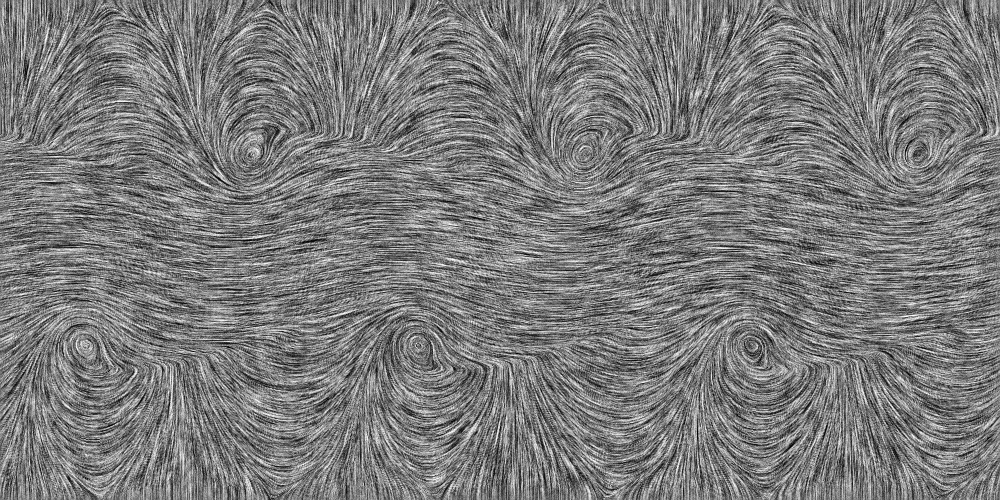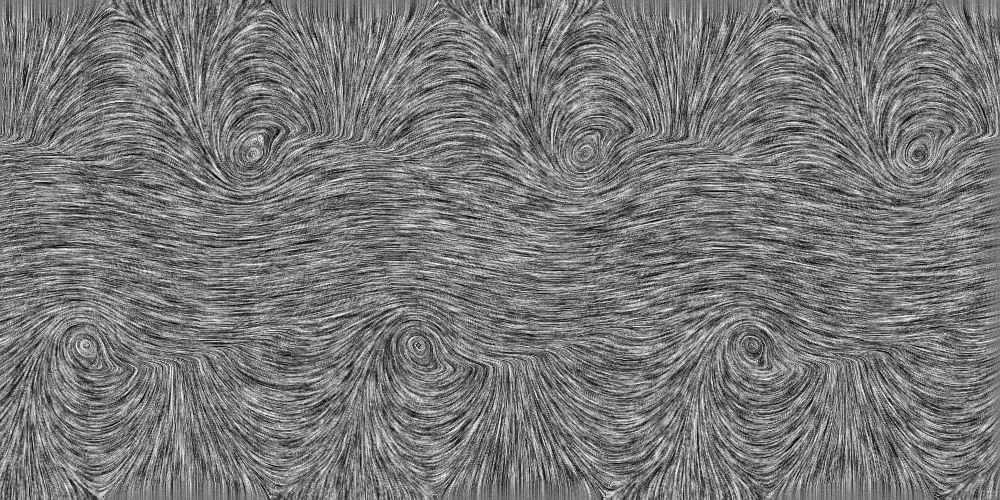# ⎣⫯ℂ: Line Integral Convolution for numpy Arrays¶This package provides line integral convolution (lic) algorithms to Python.

There are functions which can be imported and are highly configurable for the power user. Moreover, there is a command line tool to generate lic images without having to code a single line.

## Quick start¶

Install by typing

```pip install lic
```

Run the command line tool by

```lic data_x.npy data_y.npy -v -l 30 -c
```

Or use it in your program:

```import lic
import matplotlib.pyplot as plt

# ... get x and y arrays from somewhere ...

lic_result = lic.lic(x, y, length=30)

plt.imshow(lic_result, origin='lower', cmap='gray')
plt.show()
```

## What is line integral convolution?¶

Line integral convolution is a way of visualizing complete vector fields in an intuitive way.lic image of the Kelvin-Helmholtz instability

A lic image is generated by “smearing out” a random noise pattern along the flow lines of a vector field. As a result, it show the entire flow field including every detail, while the common visualizations using arrows or discrete lines will always loose fine details.

The disadvantage of (static) lic images is that the direction of the flow is not displayed. To overcome this problem, one could either overlay an array of arrows or generate a “moving” lic image. Such a moving image is generated by calculating several lic images with shifted “smearing” kernels. When animated, this gives the impression of a flow.animated lic image of the Kelvin-Helmholtz instability